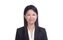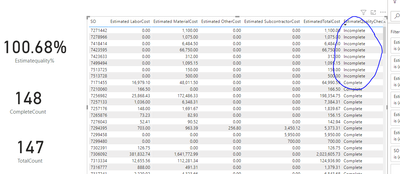cancel
Showing results for
Did you mean:Anonymous
Not applicable

## Measure Optimization - IF() Reduction

Hello - I am trying to see if I can reduce the number of steps in the following measure.  Sub Measures are as follows:

[EstimatedTotalCost] is the sum of 4 individual columns that sit in the same table.
[SumLaborCost], [SUMSubCosts], [SumMaterialCost] are each a sum of a single columns.

In summary, the measure goes through a few logic steps to determine if an estimate is a "Complete Estimate", an estimate with "Material, no Labor", or an estimate that has "No Material, No Labor".  These are determined by the correlating columns having a value >0, or =0.

The measure works as needed, but wondering if there's a more efficient way to return the desired outcome.  I am not able to add any columns to the data set, so I have to get where I'm going with a measure.

``````EstimateQuality =
var estimatedtotalcost = [EstimatedTotalCost]
var estimatedlaborcost = [SUMLaborCost]
var estimatedsubcost = [SUMSubCosts]
var estimatedmaterialcost = [SUMMaterialCost]
return
IF(
ISBLANK(estimatedtotalcost),
BLANK(),
IF(estimatedsubcost > 0,
"Complete Estimate",
IF(estimatedmaterialcost>0
&&estimatedlaborcost=0,
"Material, No Labor",
IF(estimatedtotalcost=0,"No Material, No Labor","Complete Estimate")
)
)
)``````
1 ACCEPTED SOLUTIONSuper User

Hi @Anonymous

So you go with one measure, the same test``````test =
VAR T1 =
GENERATE (
GROUPBY ( SalesHistory, SalesHistory[SO] ),
VAR EstimatedLaborCost =
CALCULATE ( SUM ( SalesHistory[Estimated LaborCost] ) )
VAR EstimatedSubCost =
CALCULATE ( SUM ( SalesHistory[Estimated SubcontractorCost] ) )
VAR EstimatedMaterialCost =
CALCULATE ( SUM ( SalesHistory[Estimated MaterialCost] ) )
VAR EstimatedOtherCost =
CALCULATE ( SUM ( SalesHistory[Estimated OtherCost] ) )
VAR EstimatedTotalCost = EstimatedLaborCost + EstimatedSubCost + EstimatedMaterialCost + EstimatedOtherCost
VAR EstimateQuality1 =
SWITCH (
TRUE (),
ISBLANK ( EstimatedTotalCost ), BLANK (),
( EstimatedTotalCost > 0
&& EstimatedMaterialCost = EstimatedTotalCost )
|| EstimatedTotalCost = 0, "Incomplete",
"Complete"
)
RETURN
ROW ( "EstimateQuality1", EstimateQuality1 )
)

VAR CompleteCount =    COUNTROWS ( FILTER ( T1, [EstimateQuality1] = "Complete" ) )
VAR TotalSO = COUNTROWS(VALUES(SalesHistory[SO]))
RETURN
DIVIDE(CompleteCount,TotalSO)``````

11 REPLIES 11Community Support

Hi @Anonymous ,

Could you tell me if your problem has been solved?
If it is, kindly Accept it as the solution. More people will benefit from it.

Best Regards,
Stephen TaoAnonymous
Not applicable

Since posting, I've gone another direction, using a combination of SWITCH() and combining all measures into multiple VARs.

To summarize, the measure is calculating total costs (EstimatedTotalCost), determining if an estimate is "Complete" or Incomplete" (Estimate Quality), Counting all estimates (CountAllEstimates), counting all complete estimates (CompleteEstimateCount), and calculating the ratio of complete to total estimates (EstimateQuality%).

In silos, each of the VARs are working as expected, except for CompleteEstimateCount. It counts all rows, not just the rows that EstaimteQuality is calling "Complete".

When I drop the EstimateQuality measure into a data table, it does call out the Sales Orders (SOs) that are and are not "Complete".

This leads me to believe that there is an issue with my COUNTAX() measure.  I have to use Distinctcount() for 'Sales History'[SO] -> There are cases where the SO has multiple service lines.  In those cases, there are two of the same SOs, but I don't want to count it as two.  Using Distinctcount() fixes that problem.

In summary, all of the VARs work in their own silos, when I try to count the "Complete" SOs, the measure counts all of the SOs, not just the ones that EstimateQuality has determined as complete.

Kudo's to the following post for getting me down this thought process.
https://community.powerbi.com/t5/Community-Blog/Performance-Tuning-DAX-Part-1/ba-p/976275

``````Estimatequality% =

VAR EstimatedLaborCost =
SUM ( SalesHistory[Estimated LaborCost] )

VAR EstimatedSubCost =
SUM ( SalesHistory[Estimated SubcontractorCost] )

VAR EstimatedMaterialCost =
SUM ( SalesHistory[Estimated MaterialCost] )

Var EstimatedOtherCost =
SUM(Sales[Estimated OtherCost])

Var EstimatedTotalCost =
SUMX (
SalesHistory,
EstimatedLaborCost + EstimatedSubCost + EstimatedMaterialCost + EstimatedOtherCost
)

Var EstimateQuality =
SWITCH(
TRUE(),
ISBLANK(EstimatedTotalCost),BLANK(),
EstimatedTotalCost > 0
&& EstimatedMaterialCost = EstimatedTotalCost
|| EstimatedTotalCost = 0 , "Incomplete",
"Complete"
)

Var CountAllEstimates =
DISTINCTCOUNT(SalesHistory[SO])

Var CompleteEstimateCount =
COUNTAX(FILTER(SalesHistory,estimatequality="Complete"),DISTINCTCOUNT(SalesHistory[SO]))

Return

DIVIDE(CompleteEstimateCount,CountAllEstimates)``````Super User

Hi @Anonymous

So you still have problem with this measure? Did not get what you want?

Can you provide some sample data with expected result? It is difficult to understand just based on a measure...

``````Var EstimateQuality =
SWITCH(
TRUE(),
ISBLANK(EstimatedTotalCost),BLANK(),
(EstimatedTotalCost > 0
&& EstimatedMaterialCost = EstimatedTotalCost)
|| EstimatedTotalCost = 0 , "Incomplete",
"Complete"
)

Var CountAllEstimates =
COUNTROWS(VALUES(SalesHistory[SO]))``````Anonymous
Not applicable

@Vera_33 Of course.  I've uploaded it to GDocs.

The table is returning what I expect in the EstimateQualityCheck measure (complete/incomplete).  However, the aggregation isn't correct in the CompleteCount measure.

I'd expect that the 8 "Incompletes" would be excluded from the CompleteCount measure.

The final calculation should return something in the ballpark of (147-8)/147 = 94.5%

In other words, why aren't these incomplete lines removing themselves from the calculation?

I also can't figure out why the CompleteCount is 148, not 147.  The Distinctcount([SalesHistory]SO) is working in the TotalCount measure.  But not when it's nested in the CompleteCount measure.Super User

Hi @Anonymous

I am running into another direction.If you are not calling a measure, but write them, wrap CALCULATE otherwise you sum up the whole table

``````test =
VAR T1 =
GENERATE (
GROUPBY ( SalesHistory, SalesHistory[SO] ),
VAR EstimatedLaborCost =
CALCULATE ( SUM ( SalesHistory[Estimated LaborCost] ) )
VAR EstimatedSubCost =
CALCULATE ( SUM ( SalesHistory[Estimated SubcontractorCost] ) )
VAR EstimatedMaterialCost =
CALCULATE ( SUM ( SalesHistory[Estimated MaterialCost] ) )
VAR EstimatedOtherCost =
CALCULATE ( SUM ( SalesHistory[Estimated OtherCost] ) )
VAR EstimatedTotalCost = EstimatedLaborCost + EstimatedSubCost + EstimatedMaterialCost + EstimatedOtherCost
VAR EstimateQuality1 =
SWITCH (
TRUE (),
ISBLANK ( EstimatedTotalCost ), BLANK (),
( EstimatedTotalCost > 0
&& EstimatedMaterialCost = EstimatedTotalCost )
|| EstimatedTotalCost = 0, "Incomplete",
"Complete"
)
RETURN
ROW ( "EstimateQuality1", EstimateQuality1 )
)
RETURN
COUNTROWS ( FILTER ( T1, [EstimateQuality1] = "Complete" ) )``````

If you do have those measures, you can simply call it

``````test1 =
VAR T1 =
ADDCOLUMNS ( VALUES ( SalesHistory[SO] ), "test111", [EstimateQualityCheck] )
RETURN
COUNTROWS ( FILTER ( T1, [test111] = "Complete" ) )``````

I am not sure how your measure was working - COUNTAX part, but it basically counts the total row of your dummy data table -  there is one SO with 2 entriesAnonymous
Not applicable

That sure does work!  Thanks so much.

One last thing.  How would you incorporate a final output of:

Divide([Test],[TotalCount]... But do it in the same measure.Super User

Hi @Anonymous

So you go with one measure, the same test``````test =
VAR T1 =
GENERATE (
GROUPBY ( SalesHistory, SalesHistory[SO] ),
VAR EstimatedLaborCost =
CALCULATE ( SUM ( SalesHistory[Estimated LaborCost] ) )
VAR EstimatedSubCost =
CALCULATE ( SUM ( SalesHistory[Estimated SubcontractorCost] ) )
VAR EstimatedMaterialCost =
CALCULATE ( SUM ( SalesHistory[Estimated MaterialCost] ) )
VAR EstimatedOtherCost =
CALCULATE ( SUM ( SalesHistory[Estimated OtherCost] ) )
VAR EstimatedTotalCost = EstimatedLaborCost + EstimatedSubCost + EstimatedMaterialCost + EstimatedOtherCost
VAR EstimateQuality1 =
SWITCH (
TRUE (),
ISBLANK ( EstimatedTotalCost ), BLANK (),
( EstimatedTotalCost > 0
&& EstimatedMaterialCost = EstimatedTotalCost )
|| EstimatedTotalCost = 0, "Incomplete",
"Complete"
)
RETURN
ROW ( "EstimateQuality1", EstimateQuality1 )
)

VAR CompleteCount =    COUNTROWS ( FILTER ( T1, [EstimateQuality1] = "Complete" ) )
VAR TotalSO = COUNTROWS(VALUES(SalesHistory[SO]))
RETURN
DIVIDE(CompleteCount,TotalSO)``````Anonymous
Not applicable

@Vera_33 That's perfect.

When incorporating with the rest of my company data, I went from ~33 seconds per operation to 8.9.  Fantastic!Super User

Hi @Anonymous

It does not matter whether the code is long or short...but the performance and the side effect... so have you tried using SWITCH instead of nested IFs? Simply change it, not sure if it is working for your logic...have a test

``````EstimateQuality =
VAR estimatedtotalcost = [EstimatedTotalCost]
VAR estimatedlaborcost = [SUMLaborCost]
VAR estimatedsubcost = [SUMSubCosts]
VAR estimatedmaterialcost = [SUMMaterialCost]
RETURN
SWITCH (
TRUE (),
ISBLANK ( estimatedtotalcost ), BLANK (),
estimatedsubcost > 0, "Complete Estimate",
estimatedmaterialcost > 0
&& estimatedlaborcost = 0, "Material, No Labor",
estimatedtotalcost = 0, "No Material, No Labor",
"Complete Estimate"
)``````

@Johanno good point to always use DAX formatter😁Responsive Resident

If it works I think you should be satisfied and move on to the next challange. 😀 There's nothing wrong using nested IF, sometimes you can avoid them by using SWITCH but SWITCH doesn't make any difference other than understanding the code since it's internally translated into nested IFs.

Sure, if you could add a column in Power Query or in the data source you might write it differently. The only thing I note is the formatting of the code (DAX Formatter is a great tool!) so it's easier to see which IFs are subordinate:

``````EstimateQuality =
VAR estimatedtotalcost = [EstimatedTotalCost]
VAR estimatedlaborcost = [SUMLaborCost]
VAR estimatedsubcost = [SUMSubCosts]
VAR estimatedmaterialcost = [SUMMaterialCost]
RETURN
IF (
ISBLANK ( estimatedtotalcost ),
BLANK (),
IF (
estimatedsubcost > 0,
"Complete Estimate",
IF (
estimatedmaterialcost > 0
&& estimatedlaborcost = 0,
"Material, No Labor",
IF ( estimatedtotalcost = 0, "No Material, No Labor", "Complete Estimate" )
)
)
)``````Anonymous
Not applicable

Thanks, @Johanno   Definetly using Dax Formatter to accomplish this nicely cascaded format 🙂